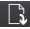# 1.12 Using the Excel Spreadsheet - Frequency Table Only

$$\newcommand{\vecs}{\overset { \rightharpoonup} {\mathbf{#1}} }$$ $$\newcommand{\vecd}{\overset{-\!-\!\rightharpoonup}{\vphantom{a}\smash {#1}}}$$$$\newcommand{\id}{\mathrm{id}}$$ $$\newcommand{\Span}{\mathrm{span}}$$ $$\newcommand{\kernel}{\mathrm{null}\,}$$ $$\newcommand{\range}{\mathrm{range}\,}$$ $$\newcommand{\RealPart}{\mathrm{Re}}$$ $$\newcommand{\ImaginaryPart}{\mathrm{Im}}$$ $$\newcommand{\Argument}{\mathrm{Arg}}$$ $$\newcommand{\norm}{\| #1 \|}$$ $$\newcommand{\inner}{\langle #1, #2 \rangle}$$ $$\newcommand{\Span}{\mathrm{span}}$$ $$\newcommand{\id}{\mathrm{id}}$$ $$\newcommand{\Span}{\mathrm{span}}$$ $$\newcommand{\kernel}{\mathrm{null}\,}$$ $$\newcommand{\range}{\mathrm{range}\,}$$ $$\newcommand{\RealPart}{\mathrm{Re}}$$ $$\newcommand{\ImaginaryPart}{\mathrm{Im}}$$ $$\newcommand{\Argument}{\mathrm{Arg}}$$ $$\newcommand{\norm}{\| #1 \|}$$ $$\newcommand{\inner}{\langle #1, #2 \rangle}$$ $$\newcommand{\Span}{\mathrm{span}}$$$$\newcommand{\AA}{\unicode[.8,0]{x212B}}$$

## Frequency Table Only

To download the Excel Workbook click on the download icon.

Click on the Frequency Table only tab on the Excel Spreadsheet below.  This spreadsheet computes the frequency table from summary data.  In the Excel spreadsheet below, you will enter the data one cell at a time.

Suppose you wanted to create a frequency table of the age of students within an Introductory Business Statistics class.  Given the data in the table below, enter the data and complete the frequency table.

Ages No. of students
15-18 3
19-22 3
23-26 9
27-30 5
31-34 7
35-38 7

Entering Values or bins in the spreadsheet

• Enter '15-18 in cell A2.
• Enter '19-22 in cell A3.
• Enter '23-26 in cell A4.
• Enter '27-30 in cell A5.
• Enter '31-34 in cell A6.
• Enter '35-38 in cell A7.

Entering the Frequency values

• Enter 3 in cell B2
• Enter 3 in cell B3.
• Enter 9 in cell B4.
• Enter 5 in cell B5.
• Enter 7 in cell B6.
• Enter 7 in cell B7.

Cumulative Frequency (Cum. Freq.is a running total of the frequency values.

Relative Frequency (Rel. Freq.) is the frequency value divided by the total frequency.

Cumulative Relative Frequency (Cum. Rel. Freqis the cumulative frequency divided by the total frequency.

Ages No. of students Cum. Freq. Rel. Freq. Cum. Rel. Freq
15-18 3 3

=3/34
0.0882

=3/34=0.0882
19-22 3 =3+3 = 6 =3/34
=0.0882
=6/34=0.1765
23-26 9 =6 + 9 =15 =9/34
0.2647
=15/34=0.4412
27-30 5 =15+5 = 20 =5/34
0.1471
=20/34=0.5882
31-34 7 =20+7 = 27 =7/34
0.2059
=27/34=0.7941
35-38 7 =27+7 = 34 =7/34
0.2059
=34/34=1
34

Were there are blue cells in the Excel spreadsheet, enter the information above.

Completing the Table

• Enter 3 in cell C2.
• Enter 27 in cell C6.
• Enter 0.2647 in cell D4.
• Enter 0.2059 in cell D7.
• Enter 0.0882 in cel E2.
• Enter 0.5882 in cell E5.
• Enter 0.7941 in cell E6.

1.12 Using the Excel Spreadsheet - Frequency Table Only is shared under a not declared license and was authored, remixed, and/or curated by LibreTexts.